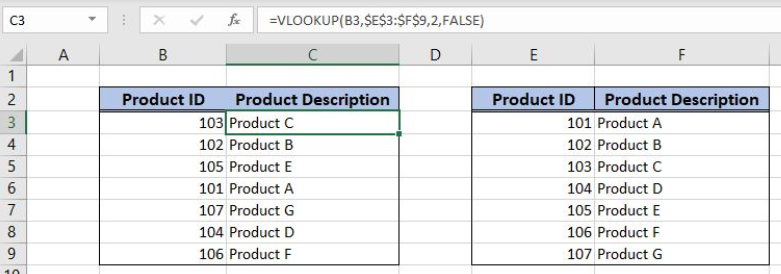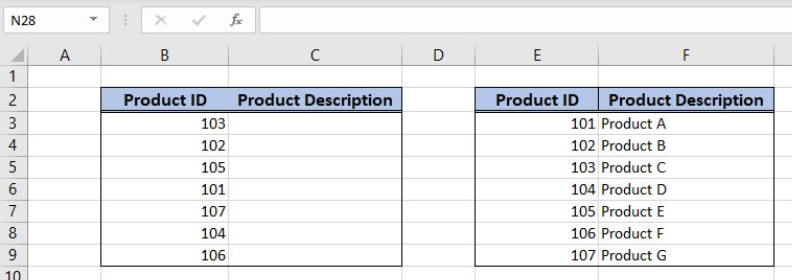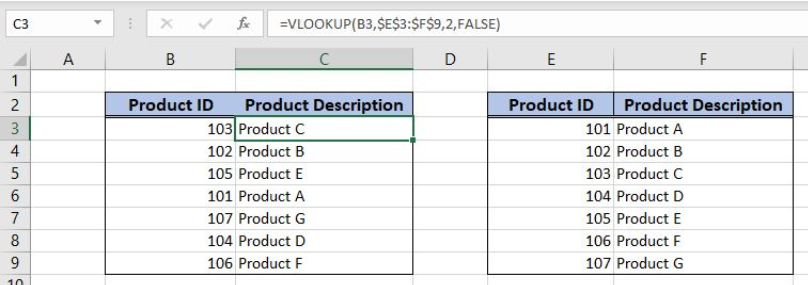Get instant live expert help with Excel or Google Sheets“My Excelchat expert helped me in less than 20 minutes, saving me what would have been 5 hours of work!”

#### Post your problem and you’ll get expert help in seconds.

Your message must be at least 40 characters
Our professional experts are available now. Your privacy is guaranteed.

# How to Use the table_array Argument in an Excel Lookup Function

Excel allows us to lookup values from a table_array using the VLOOKUP function. This step by step tutorial will assist all levels of Excel users in using the table_array argument in an Excel VLOOKUP function.Figure 1. The final result of the formula

## Syntax of the VLOOKUP formula

The generic formula for the VLOOKUP function is:

`=VLOOKUP(lookup_value, table_array, col_index_num, range_lookup)`

The parameters of the VLOOKUP function are:

• lookup_value – a value that we want to find in a table_array
• table_array – a table range in which we want to look up for a value
• col_index_num – a column number in table_array from which we would like to get a value
• range_lookup – default value is FALSE. This means that we want to find an exact match for a lookup value.

## Setting up Our Data for the Example

Our tables consist of 2 columns: “Product ID” and “Product Description”. In column C, we want to get a Product description from the column F based on Product ID match. In this case, our table_array is the range E3:F9.Figure 2. Data that we will use in the example

## Using the VLOOKUP Formula

We want to get a product description in the cell C3, from the table_array E3:F9, based on the Product ID 103 in the cell B3.

The formula looks like:

`=VLOOKUP(B3, \$E\$3:\$F\$9, 2, FALSE)`

The lookup_value parameter is B3. The table_array is \$E\$3:\$F\$9. The range must be fixed, as it’s not changing when the formula is copied to the other cells. The col_index_num is 2 because we want to get a value from the second column. The range_lookup is FALSE.

To apply the VLOOKUP formula, we need to follow these steps:

• Select cell C3 and click on it
• Insert the formula: `=VLOOKUP(B3, \$E\$3:\$F\$9, 2, FALSE)`
• Press enter
• Drag the formula down to the other cells in the column by clicking and dragging the little “+” icon at the bottom-right of the cell.Figure 3. Using the VLOOKUP formula

The function returns “Product C” in F3 as this is the description for the Product ID 103 from C3 in the table_array.

Most of the time, the problem you will need to solve will be more complex than a simple application of a formula or function. If you want to save hours of research and frustration, try our live Excelchat service! Our Excel Experts are available 24/7 to answer any Excel question you may have. We guarantee a connection within 30 seconds and a customized solution within 20 minutes.

Solution examplesI have a pivot table in which per order (on the rows) stands how much products they ordered per size (on the columns). I want to determine which combinations of quantities of sizes people order. And I want to count these combinations.
Solved by E. W. in 60 minsI have 500 numbers in column A with aproximatly 15digits. In column B I have 5 numbers with 6 digits Can a find a formula wich can transfer all numbers from A column wich start with some 6 digitsa from B column
Solved by V. L. in 32 minsI filtered data in column A (it is labeled ID #) of a data set. On my next worksheet, column A is also the ID #, but it is a different data set. I want to filter the ID #'s the same for the two sheets, but how do I do that being that I am working with two different sets of data?
Solved by V. H. in 22 minsI have a sheet with 3 columns. First column is a code for records in column B (has 563 records). What I need is a formula to show me if what is in column C (has 4400 records) is in column B and if it is to take the code for that record. Example: Column A(code corresponding to column B): 12, 14, 15, 19 Column B(names): asd, adf, ade, aqw Column C(names): akd, adf, ade, anb, wgs I need something that would say, if record in column C (for example adf) is matching record in column B (adf) return the code from column A (the code corresponding to that record in column B),if not return blank.
Solved by C. J. in 8 minsIf the data in A matches the data in C, then I need the data in E to be placed in B
Solved by X. W. in 40 mins